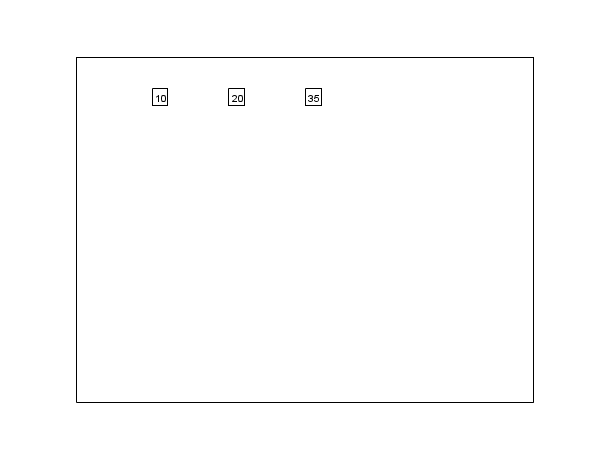# xnumb

draw numbers

### Syntax

`xnumb(x, y, nums, [box, angle])`

### Arguments

x, y, nums

vectors of same size.

box

an integer value.

angle

an optional vector of same size as `x`

### Description

`xnumb` draws the value of `nums(i)` at position `x(i), y(i)` in the current scale. If `box` is 1, a box is drawn around the numbers. If `angle` is given, it gives the direction for string drawing.Note that a maximum of 4 significant digits of each element of `nums` will be displayed.

### Examples

```plot2d([-100,500],[-100,600],[-1,-1],"022")
x=0:100:200;
xnumb(x,500*ones(x),[10,20,35],1)```